## ML Aggarwal Class 10 Solutions for ICSE Maths Chapter 5 Quadratic Equations in One Variable Ex 5.2

These Solutions are part of ML Aggarwal Class 10 Solutions for ICSE Maths. Here we have given ML Aggarwal Class 10 Solutions for ICSE Maths Chapter 5 Quadratic Equations in One Variable Ex 5.2

More Exercises

Solve the following equations (1 to 24) by factorization

Question 1.
(i) 4x² = 3x
(ii) $$\frac { { x }^{ 2 }-5x }{ 2 } =0$$
Solution:
(i) 4x² = 3x
x(4x – 3) = 0
Either x = 0,Question 2.
(i) (x – 3) (2x + 5) = 0
(ii) x (2x + 1) = 6
Solution:
(i) (x – 3) (2x + 5) = 0
Either x – 3 = 0,
Then x = 3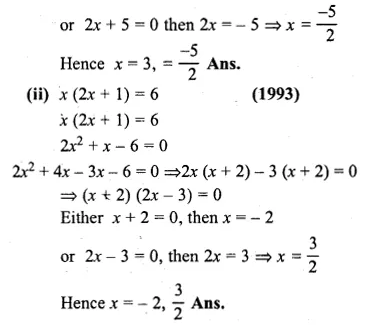Question 3.
(i) x² – 3x – 10 = 0
(ii) x(2x + 5) = 3
Solution:
(i) x² – 3x – 10 = 0
⇒ x² – 5x + 2x – 10 = 0
⇒ x(x – 5) + 2(x – 5) = 0Question 4.
(i) 3x² – 5x – 12 = 0
(ii) 21x² – 8x – 4 = 0
Solution:
(i) 3x² – 5x – 12 = 0
⇒ 3x² – 9x + 4x – 12 = 0
⇒ 3x (x – 3) + 4(x – 3) = 0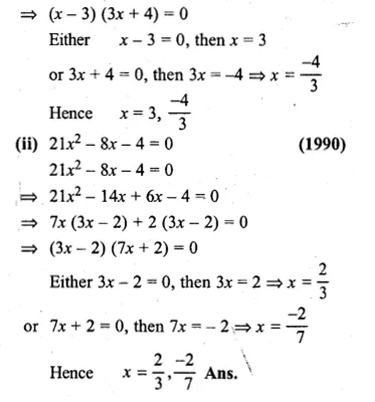Question 5.
(i) 3x² = x + 4
(ii) x(6x – 1) = 35
Solution:
(i) 3x² = x + 4
⇒ 3x² – x – 4 = 0
⇒ 3x² – 4x + 3x – 4 = 0Question 6.
(i) 6p² + 11p – 10 = 0
(ii) $$\frac { 2 }{ 3 } { x }^{ 2 }-\frac { 1 }{ 3 } x=1$$
Solution:
(i) 6p² + 11p – 10 = 0
⇒ 6p² + 15p – 4p – 10 = 0
⇒ 3p(2p + 5) – 2(2p + 5) = 0Question 7.
(i) (x – 4)² + 5² = 13²
(ii) 3(x – 2)² = 147
Solution:
(i) (x – 4)² + 5² = 13²
x² – 8x + 16 + 25 = 169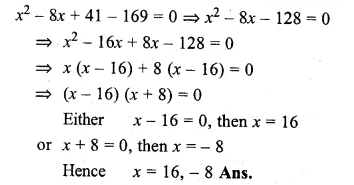Question 8.
(i) $$\\ \frac { 1 }{ 7 }$$(3x – 5)² = 28
(ii) 3(y² – 6) = y(y + 7) – 3
Solution:
(i) $$\\ \frac { 1 }{ 7 }$$(3x – 5)² = 28
(3x – 5)² = 28 × 7
⇒ 9x² – 30x + 25 = 196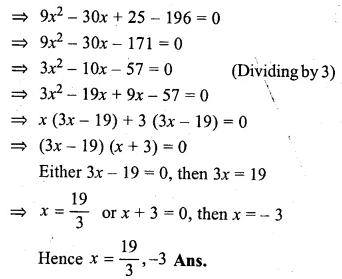Question 9.
x² – 4x – 12 = 0,when x∈N
Solution:
x² – 4x – 12 = 0
⇒ x² – 6x + 2x – 12 = 0
⇒ x (x – 6) + 2 (x – 6) = 0
⇒ (x – 6) (x + 2) = 0
Either x – 6 = 0, then x = 6
or x + 2 = 0, then x = -2
But -2 is not a natural number
∴ x = 6

Question 10.
2x² – 8x – 24 = 0 when x∈I
Solution:
2x² – 8x – 24 = 0
⇒ x² – 4x – 12 = 0 (Dividing by 2)
⇒ x² – 6x + 2x – 12 = 0
⇒ x (x – 6) + 2 (x – 6) = 0
⇒ (x – 6) (x + 2) = 0
Either x – 6 = 0, then, x = 6
or x + 2 = 0, then x = – 2
Hence x = 6, – 2

Question 11.
5x² – 8x – 4 = 0 when x∈Q
Solution:
5x² – 8x – 4 = 0
∵ 5 × ( – 4) = – 20
-20 = – 10 + 2
-8 = – 10 + 2Question 12.
2x² – 9x + 10 = 0,when
(i)x∈N
(ii)x∈Q
Solution:
2x² – 9x + 10 = 0
⇒ 2x² – 4x – 5x + 10 = 0
⇒ 2x(x – 2) – 5(x – 2) = 0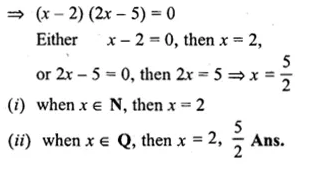Question 13.
(i) a²x² + 2ax + 1 = 0, a≠0
(ii) x² – (p + q)x + pq = 0
Solution:
(i) a²x² + 2ax + 1 = 0
⇒ a²x² + ax + ax + 1 = 0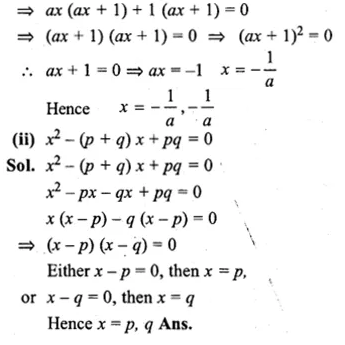Question 14.
a²x² + (a² + b²)x + b² = 0, a≠0
Solution:
a²x² + (a² + b²)x + b² = 0
⇒ a²x(x + 1) + b²(x + 1) = 0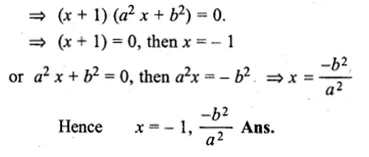Question 15.
(i) √3x² + 10x + 7√3 = 0
(ii) 4√3x² + 5x – 2√3 = 0
Solution:
(i) √3x² + 10x + 7√3 = 0
[ ∵ √3 x 7√3 = 7 x 3 = 21]
⇒ √3x(x + √3) + 7(x + √3) = 0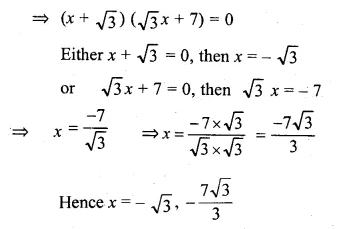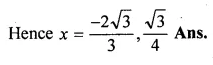Question 16.
(i) x² – (1 + √2)x + √2 = 0
(ii) $$x+ \frac { 1 }{ x }$$ = $$2 \frac { 1 }{ 20 }$$
Solution:
(i) x² – (1 + √2)x + √2 = 0
⇒ x² – x – √2x + √2 = 0Question 17.
(i) $$\frac { 2 }{ { x }^{ 2 } } -\frac { 5 }{ x } +2=0,x\neq 0$$
(ii)$$\frac { { x }^{ 2 } }{ 15 } -\frac { x }{ 3 } -10=0$$
Solution:
(i) $$\frac { 2 }{ { x }^{ 2 } } -\frac { 5 }{ x } +2=0,x\neq 0$$
⇒ 2 – 5x + 2x² = 0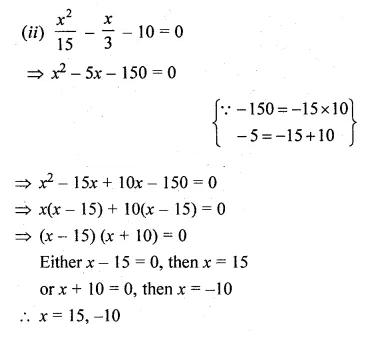Question 18.
(i) $$3x-\frac { 8 }{ x } =2$$
(ii) $$\frac { x+2 }{ x+3 } =\frac { 2x-3 }{ 3x-7 }$$
Solution:
(i) $$3x-\frac { 8 }{ x } =2$$
$$\frac { { 3x }^{ 2 }-8 }{ x } =2$$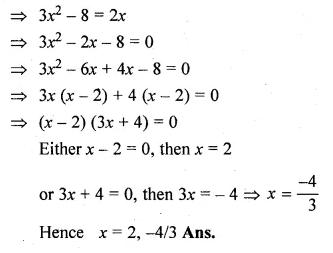Question 19.
(i) $$\frac { 8 }{ x+3 } -\frac { 3 }{ 2-x } =2$$
(ii) $$\frac { x }{ x-1 } +\frac { x-1 }{ x } =2\frac { 1 }{ 2 }$$
Solution:
(i) $$\frac { 8 }{ x+3 } -\frac { 3 }{ 2-x } =2$$
$$\frac { 16-8x-3x-9 }{ (x+3)(2-x) } =2$$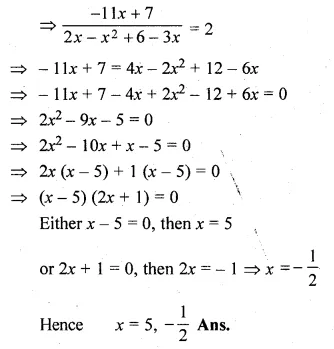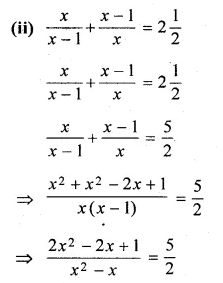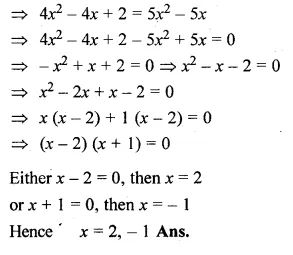Question 20.
(i) $$\frac { x }{ x+1 } +\frac { x+1 }{ x } =\frac { 34 }{ 15 }$$
(ii) $$\frac { x+1 }{ x-1 } +\frac { x-2 }{ x+2 } =3$$
Solution:
(i) $$\frac { x }{ x+1 } +\frac { x+1 }{ x } =\frac { 34 }{ 15 }$$
$$\frac { { x }^{ 2 }+{ x }^{ 2 }+2x+1 }{ x(x+1) } =\frac { 34 }{ 15 }$$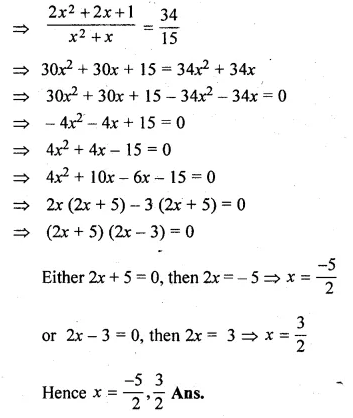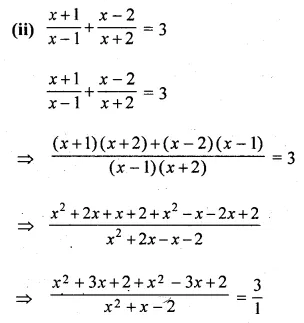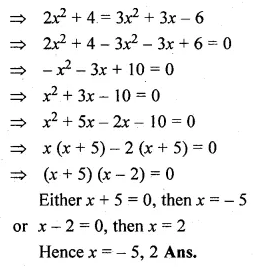Question 21.
(i) $$\frac { 1 }{ x-3 } -\frac { 1 }{ x+5 } =\frac { 1 }{ 6 }$$
(ii) $$\frac { x-3 }{ x+3 } +\frac { x+3 }{ x-3 } =2\frac { 1 }{ 2 }$$
Solution:
(i) $$\frac { 1 }{ x-3 } -\frac { 1 }{ x+5 } =\frac { 1 }{ 6 }$$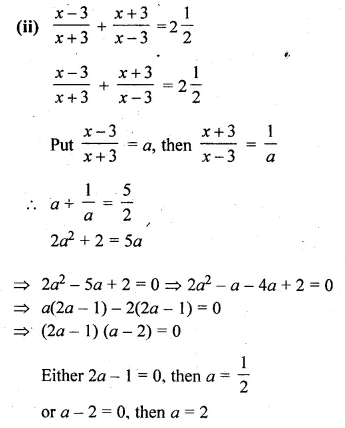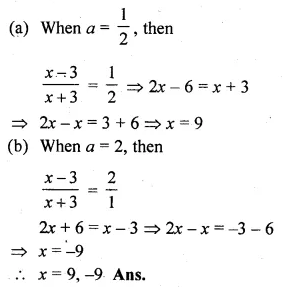Question 22.
(i) $$\frac { a }{ ax-1 } +\frac { b }{ bx-1 } =a+b,a+b\neq 0,ab\neq 0$$
(ii) $$\frac { 1 }{ 2a+b+2x } =\frac { 1 }{ 2a } +\frac { 1 }{ b } +\frac { 1 }{ 2x }$$
Solution:
(i) $$\frac { a }{ ax-1 } +\frac { b }{ bx-1 } =a+b$$
⇒ $$\left( \frac { a }{ ax-1 } -b \right) +\left( \frac { b }{ bx-1 } -a \right) =0$$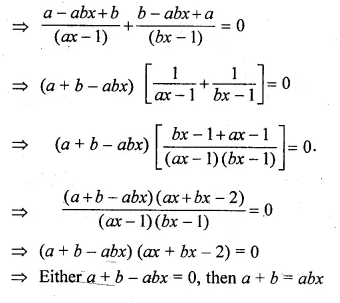Question 23.
$$\frac { 1 }{ x+6 } +\frac { 1 }{ x-10 } =\frac { 3 }{ x-4 }$$
Solution:
$$\frac { 1 }{ x+6 } +\frac { 1 }{ x-10 } =\frac { 3 }{ x-4 }$$
⇒ $$\frac { x-10+x+6 }{ (x+6)(x-10) } =\frac { 3 }{ x-4 }$$Question 24.
(i) $$\sqrt { 3x+4 } =x$$
(ii) $$\sqrt { x(x-7) } =3\sqrt { 2 }$$
Solution:
(i) $$\sqrt { 3x+4 } =x$$
Squaring on both sides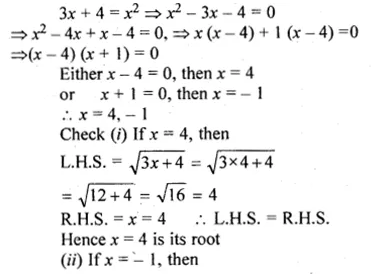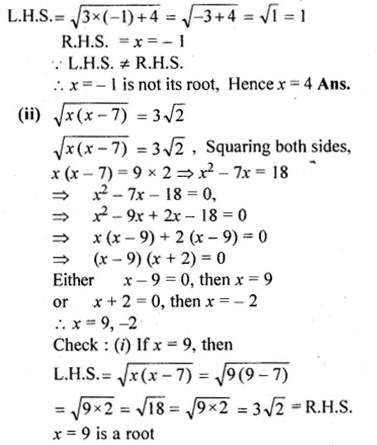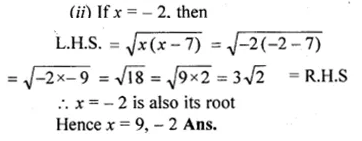Question 25.
Use the substitution y = 3x + 1 to solve for x : 5(3x + 1 )² + 6(3x + 1) – 8 = 0
Solution:
y = 3x + 1
Now, 5(3x + 1)² + 6(3x + 1) – 8 = 0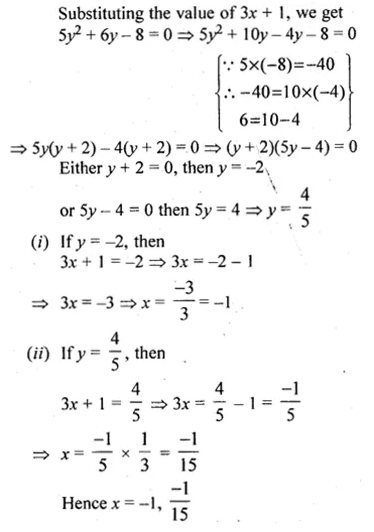Question 26.
Find the values of x if p + 1 =0 and x² + px – 6 = 0
Solution:
p + 1 = 0, then p = – 1
Substituting the value of p in the given quadratic equation
x² + ( – 1)x – 6 = 0
⇒ x² – x – 6 = 0
⇒ x² – 3x + 2x – 6 = 0
⇒ x (x – 3) + 2 (x – 3) = 0
⇒ (x – 3) (x + 2) = 0
Either x – 3 = 0, then x = 3
or x + 2 = 0, then x = – 2
Hence x = 3, -2

Question 27.
Find the values of x if p + 7 = 0, q – 12 = 0 and x² + px + q = 0,
Solution:
p + 7 = 0, then p = – 7
and q – 12 = 0, then q = 12
Substituting the values of p and q in the given quadratic equation,
x² – 7x + 12 = 0
⇒ x² – 3x – 4x + 12 = 0
⇒ x (x – 3) – 4 (x – 3) = 0
⇒ (x – 3) (x – 4) = 0
Either x – 3 = 0, then x = 3
or x – 4 = 0, then x = 4
Hence x = 3, 4

Question 28.
If x = p is a solution of the equation x(2x + 5) = 3, then find the value of p.
Solution:
Given, x = p and x(2x + 5) = 3
Substituting the value of p, we get
p(2p + 5) = 3
⇒ 2p² + 5p – 3 = 0
⇒ 2p² + 6p – p – 3 = 0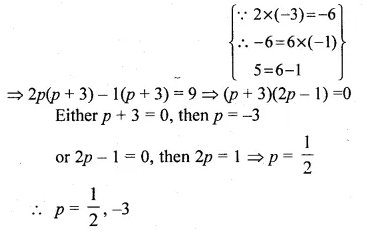Hope given ML Aggarwal Class 10 Solutions for ICSE Maths Chapter 5 Quadratic Equations in One Variable Ex 5.2 are helpful to complete your math homework.

If you have any doubts, please comment below. Learn Insta try to provide online math tutoring for you.